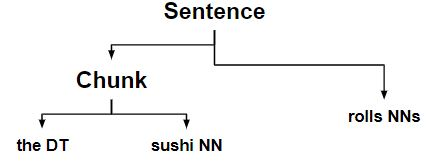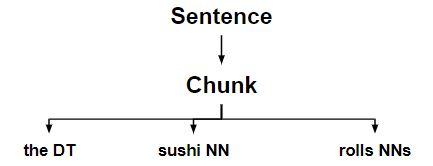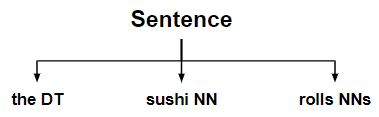Related Articles
NLP | Expanding and Removing Chunks with RegEx
• Last Updated : 29 Jan, 2019

RegexpParser or RegexpChunkRule.fromstring() doesn’t support all the `RegexpChunkRule classes`. So, we need to create them manually.

ExpandRightRule: It adds chink (unchunked) words to the right of a chunk.
ExpandLeftRule: It adds chink (unchunked) words to the left of a chunk.
For `ExpandLeftRule `and `ExpandRightRule `takes as parameter – the right and left chink pattern respectively that we want to add to the beginning and ending of the chunk respectively.

UnChunkRule: It unchunks any matching chunk and it becomes a chink.

Code #1: How the code works

 `# Loading Libraries``from` `nltk.chunk.regexp ``import` `ChunkRule, ExpandLeftRule``from` `nltk.chunk.regexp ``import` `ExpandRightRule, UnChunkRule``from` `nltk.chunk ``import` `RegexpChunkParser`` ` `# Initialising ChunkRule``ur ``=` `ChunkRule(``''``, ``'single noun'``)`` ` `# Initialising ExpandLeftRule``el ``=` `ExpandLeftRule(``'
'``, ``''``, ``'get left determiner'``)`` ` `# Initialising ExpandRightRule``er ``=` `ExpandRightRule(``''``, ``''``, ``'get right plural noun'``)`` ` `# Initialising UnChunkRule``un ``=` `UnChunkRule(``'
*'``, ``'unchunk everything'``)`` ` `chunker ``=` `RegexpChunkParser([ur, el, er, un])`` ` `sent ``=` `[(``'the'``, ``'DT'``), (``'sushi'``, ``'NN'``), (``'rolls'``, ``'NNS'``)]`` ` `chunker.parse(sent)`

Output:

`Tree('S', [('the', 'DT'), ('sushi', 'NN'), ('rolls', 'NNS')])`

Note: Output is a flat sentence as UnChunkRule undid the chunk created by the previous rules.

How the stuff works?

• Make a chunk with noun.• Expanding the left determiners to chunks that begin with noun.• Expanding the right plural nouns to chunks that ends with noun.• Finally, it unchunk every chunk that is a determiner + noun + plural noun, resulting in the original sentence tree.Code #2: Step by Step Code Explaining the diagram.

 `# Loading Libraries``from` `nltk.chunk.regexp ``import` `ChunkRule, ExpandLeftRule``from` `nltk.chunk.regexp ``import` `ExpandRightRule, UnChunkRule``from` `nltk.chunk ``import` `RegexpChunkParser``from` `nltk.chunk.regexp ``import` `ChunkString``from` `nltk.tree ``import` `Tree`` ` `chunk_string ``=` `ChunkString(Tree(``'S'``, sent))``print` `(``"Chunk String : "``, chunk_string)`` ` `# Initialising ChunkRule``ur ``=` `ChunkRule(``''``, ``'single noun'``)``ur.``apply``(chunk_string)``print` `(``"\nstep 1 : "``, chunk_string)`` ` `# Initialising ExpandLeftRule``el ``=` `ExpandLeftRule(``'
'``, ``''``, ``'get left determiner'``)``el.``apply``(chunk_string)``print` `(``"step 2 : "``, chunk_string)`` ` `# Initialising ExpandRightRule``er ``=` `ExpandRightRule(``''``, ``''``, ``'get right plural noun'``)``er.``apply``(chunk_string)``print` `(``"step 3 : "``, chunk_string)`` ` `# Initialising UnChunkRule``un ``=` `UnChunkRule(``'
*'``, ``'unchunk everything'``)``un.``apply``(chunk_string)``print` `(``"step 4 : "``, chunk_string)`

Output :

```Chunk String :   <DT>  <NN>  <NNS>

step 1 :   <DT> {<NN>} <NNS>
step 2 :  {<DT>  <NN>} <NNS>
step 3 :  {<DT>  <NN>  <NNS>}
step 4 :   <DT>  <NN>  <NNS>```

Attention geek! Strengthen your foundations with the Python Programming Foundation Course and learn the basics.

To begin with, your interview preparations Enhance your Data Structures concepts with the Python DS Course. And to begin with your Machine Learning Journey, join the Machine Learning – Basic Level Course

My Personal Notes arrow_drop_up